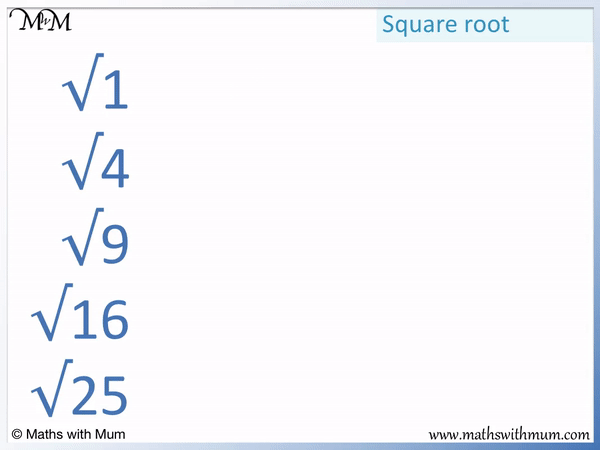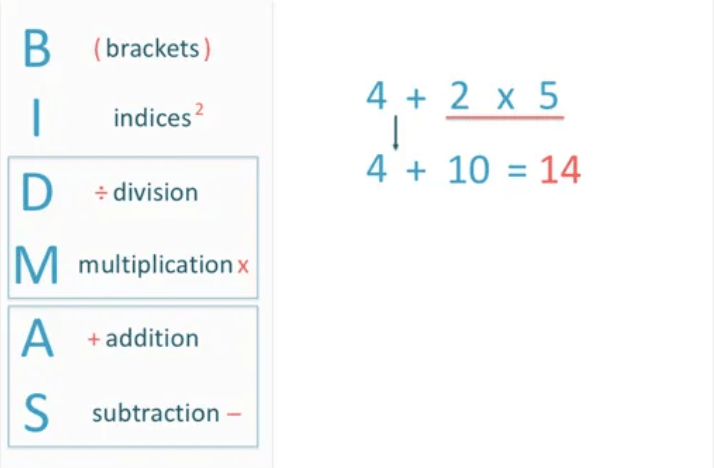# How to Find the Square Root of a Number

How to Find the Square Root of a Number• To find the square root of a number, think “What number multiplied by itself gives us this number?”
• A square root of a number multiplied by itself makes that number.
• The square root of a whole number is smaller than the original number.
• The mathematical symbol √ means to find the square root of the number that comes after it.
• The square root of 1 is 1 because 1 × 1 = 1.
• The square root of 4 is 2 because 2 × 2 = 4.
• The square root of 9 is 3 because 3 × 3 = 9.
• The square root of 16 is 4 because 4 × 4 = 16.
• The square root of 25 is 5 because 5 × 5 = 25.
The square root of a given number is a number that is multiplied by itself to equal this given number.

#### List of Square Roots• To find the square root of a number, find the number that can be multiplied by itself to make it.
• 5 × 5 = 25 and so, the square root of 25 is 5.
• √25 means to find the square root of 25.
• We can write √25 = 5.
• We can make a square that is 5 cm long. Its area is 25 cm2.
• The side length of a square is the square root of the area of that square.Supporting Lessons#### Square Root Calculator

Enter a number to calculate its square root:

# Square Roots

## What is a Square Root?

The square root of a number is another number that when multiplied by itself equals this number. For example, the square root of 25 is 5 because 5 × 5 = 25.

Instead of writing ‘find the square root of’ in front of each number, it is quicker to use the square root sign.The mathematical symbol for finding the square root is √. It is written immediately before the number that is to be square rooted. For example, √36 means to find the square root of 36. It means to find the number that when multiplied by itself equals 36. √36 = 6 because 6 × 6 = 36.

Here is a list of square roots:

Number Square Root
1 1
4 2
9 3
16 4
25 5
36 6
49 7
64 8
81 9
100 10
121 11
144 12

Here is a downloadable, printable poster showing the first 12 whole number square roots.

Square roots are called square roots because any square has a side length that is equal to the square root of the area. The relationship between a number and its square root can be shown using a square. The word root simply means a solution to an equation.

For example, here is a square of area 25.

The area of a square is the length of one of the sides multiplied by itself.The length of each side of the square is 5. The area of the square is 5 × 5 = 25.

When a number is multiplied by itself we say that the number has been squared.

We say that 5 squared is 25.

Finding the square root is the opposite of squaring a number. They are inverse functions. Squaring means to multiply a number by itself. Finding the square root means to find the number that can be multiplied by itself to give that number.

Since we know that 5 × 5 = 25, we know that 5 is the square root of 25.The square root of a given number can be thought of as the side length of a square which has an area equal to that given number.

## How to Find the Square Root of a Number

To find the square root of a given number, find the number that can be multiplied by itself to equal the given number. Knowing the times tables is helpful for finding the square roots more quickly.

A perfect square root is a square root that is a whole number.

Here is a list of perfect square roots:

• √1 = 1.
• √4 = 2.
• √9 = 3.
• √16 = 4.
• √25 = 5.
• √36 = 6.
• √49 = 7.
• √64 = 8.
• √81 = 9.
• √100 = 10.
• √121 = 11.
• √144 = 12.Perfect square roots are the square roots of square numbers. They are always whole numbers.

When introducing the idea of square roots, it is best to start with finding the square roots of perfect squares. Start by teaching the square numbers by multiplying a number by itself. Use these square number facts to then work out the square roots.

You can find the square root of all numbers except for negative numbers. Negative numbers do not have a square root. This is because when a number is multiplied by itself, the answer is always positive. Even a negative number multiplied by itself makes a positive answer because two negatives multiplied together cancel out.

The square root of 0 is 0 because 0 × 0 = 0.

To find the square roots of non perfect squares, the easiest and most common method is to use a calculator. Simply press the √ key, type the number and press the = key.

There is a square root calculator on this page which can be used to find the square roots of non perfect squares.

The square roots of non perfect squares will be between the square roots of the perfect squares either side of the number.

For example, √9 = 3 and √4 = 2 so the square root of 5 must be less than 3 but larger than 2. The square root of 5 is actually 2.2360679775, which is less than 3 but larger than 2.Now try our lesson on How to Use BODMAS (BIDMAS/PEMDAS) where we learn what order to do a calculation in.error: Content is protected !!